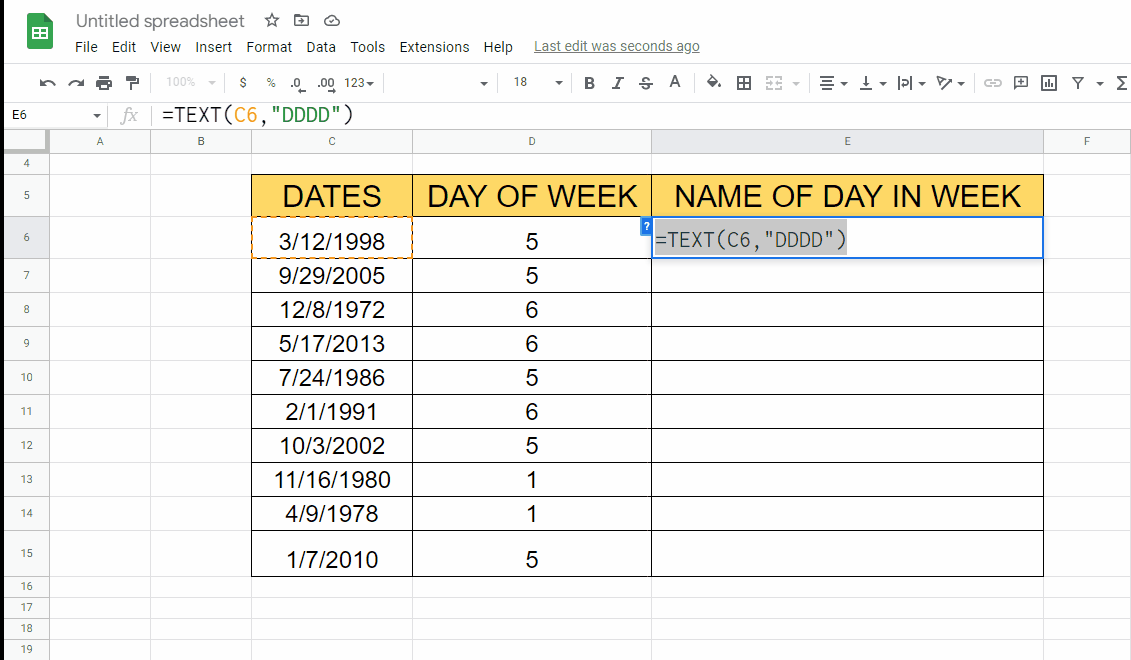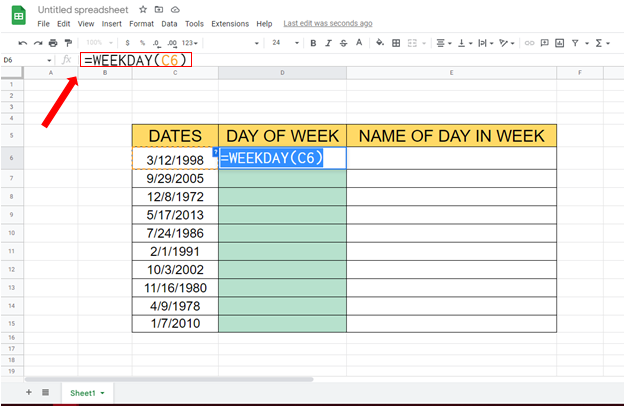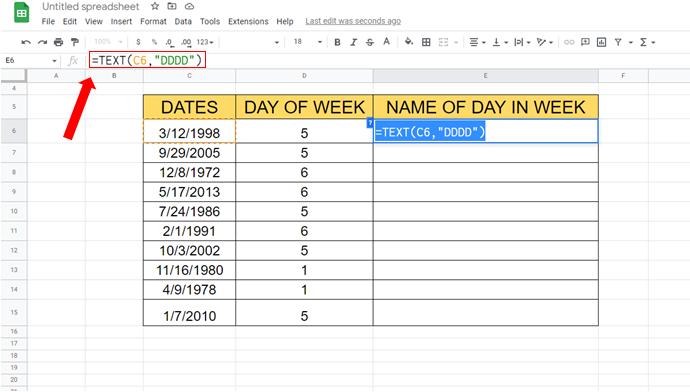# How to find days of week using Google SheetsThe dataset we are using contains 3 columns, First column is for DATES, Second column is for DAYS OF WEEK in numbers and third column is for NAMES OF DAYS IN WEEK. Two different formulas will be used in this tutorial, step by step procedure is given below. First of all let’s have a look at the dataset above. First column is for Dates (MM/DD/YYYY), Second column is for DAY OF WEEK (1 – 7) in numbers and the third column is for NAME OF DAY IN WEEK (Monday, Tuesday, etc)Google Sheets is a powerful tool for managing and analyzing data, and one area where it excels is in working with dates. In this tutorial, we’ll explore how to use Google Sheets to work with days of the week.

### Step 1 – Find DAY OF WEEK.– To find day of week from date we will use WEEKDAY() formula, Syntax for this formula will be:
=WEEKDAY( Value )
– In our case WEEKDAY() formula will look like this:
=WEEKDAY(C6)

### Step 2 – Find DAY OF WEEK for the remaining cells.– Drag the cell with the formula from bottom right to the remaining cells to find their value automatically.

### Step 3 – Find NAME OF DAY IN WEEK.– For the name of the day of the week we’ll be using TEXT() Formula.
– Syntax of this formula will be
=TEXT(Value, ”Format”)
– In our case formula will be
=TEXT(C6, ”DDDD”)

### Step 4 – Find NAME OF DAY IN WEEK for remaining cells.– Drag the cell with the formula from bottom right to the other cells to find remaining values automatically.